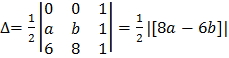# The vertices of a family of triangles have integer coordinates. If two of the vertices of all the triangles are (0, 0) and (6, 8), then the least value of areas of the triangles is a) 1 b)c) 2 d)## Question ID - 50347 :- The vertices of a family of triangles have integer coordinates. If two of the vertices of all the triangles are (0, 0) and (6, 8), then the least value of areas of the triangles is a) 1 b)c) 2 d)3537

(a)

Let the third vertex beArea ofAsare integers, so we take

(0, 0), (1, 1), (1, 2)

At, it is not possible

AtAtHere, we see that minimum area is 1

Next Question :
 In ais equal to a)b)c)d)